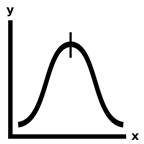# Exercise #14: Average

average([12, 20, 37])   23Averages are an essential statistical tool, and computers make it easy to calculate the average of millions or billions of numbers. The average is the sum of a set of the numbers divided by the amount of numbers. For example, the average of 12, 1, and 5 is 6, because 12 + 1 + 5 is 18 and 18 / 3 is 6. This and the following two exercises challenge you to make Python solve these statistics calculations.

Exercise Description

Write an `average()` function that has a `numbers` parameter. This function returns the statistical average of the list of integer and floating-point numbers passed to the function. While Python’s built-in `sum()` function can help you solve this exercise, try writing the solution without using it.

Passing an empty list to `average()` should cause it to return `None`.

These Python `assert` statements stop the program if their condition is `False`. Copy them to the bottom of your solution program. Your solution is correct if the following `assert` statements’ conditions are all True:

assert average([1, 2, 3]) == 2

assert average([1, 2, 3, 1, 2, 3, 1, 2, 3]) == 2

assert average([12, 20, 37]) == 23

assert average([0, 0, 0, 0, 0]) == 0

import random

random.seed(42)

testData = [1, 2, 3, 1, 2, 3, 1, 2, 3]

for i in range(1000):

random.shuffle(testData)

assert average(testData) == 2

Shuffling the order of the numbers should not affect the average. The `for` loop does 1,000 such random shuffles to thoroughly check that this fact remains true. For an explanation of the random.seed() function, see the Further Reading section of Exercise #19, “Password Generator”.

Try to write a solution based on the information in this description. If you still have trouble solving this exercise, read the Solution Design and Special Cases and Gotchas sections for additional hints.

Prerequisite concepts: `len()`, for loops, augmented assignment operators

Solution Design

Create a variable that holds the sum total of the numbers in the numbers list. Then write a `for` loop to go over all the numbers in the list, adding them to this total. This is identical to the `calculateSum()` function in Exercise #13 “Sum & Product.” After the loop, return the total divided by the amount of numbers in the `numbers` list. You can use Python’s built-in `len()` function for this.

While you shouldn’t use Python’s built-in `sum()` function, you can import your sumproduct.py program from Exercise #13, “Sum & Product” for its `calculateSum()` function. After completing Exercise #13, make sure sumproduct.py is in the same folder as average.py. Then use `import sumproduct` to import the module and sumproduct.calculateSum() to call the function.

Special Cases and Gotchas

If the `numbers` parameter is an empty list, the function should return `None`. Therefore, you should put the code that checks this at the start of the function.

Now try to write a solution based on the information in the previous sections. If you still have trouble solving this exercise, read the Solution Template section for additional hints.

Solution Template

Try to first write a solution from scratch. But if you have difficulty, you can use the following partial program as a starting place. Copy the following code from https://invpy.com/average-template.py and paste it into your code editor. Replace the underscores with code to make a working program:

def average(numbers):

# Special case: If the numbers list is empty, return None:

if len(____) == ____:

return ____

# Start the total at 0:

total = ____

# Loop over each number in numbers:

for number in ____:

# Add the number to the total:

total ____ number

# Get the average by dividing the total by how many numbers there are:

return ____ / ____(numbers)

The complete solution for this exercise is given in Appendix A and https://invpy.com/average.py. You can view each step of this program as it runs under a debugger at https://invpy.com/average-debug/.